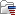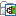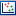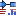## PCA for RNA-Seq

Principal Component Analysis makes it possible to project a high-dimensional dataset (where the number of dimensions equals the number of genes or transcripts) onto two or three dimensions. This helps in identifying outlying samples for quality control, and gives a feeling for the principal causes of variation in a dataset. The analysis proceeds by transforming a large set of variables (in this case, the counts for each individual gene or transcript) to a smaller set of orthogonal principal components. The first principal component specifies the direction with the largest variability in the data, the second component is the direction with the second largest variation, and so on.

The PCA for RNA-Seq tool clusters samples in 2D or 3D. Known metadata about each sample is added as an overlay. In addition, the following filtering and normalization are performed:

• 'log CPM' (Counts per Million) values are calculated for each gene. The CPM calculation uses the effective library sizes as calculated by the TMM normalization.
• After this, a Z-score normalization is performed across samples for each gene: the counts for each gene are mean centered, and scaled to unit variance.
• Genes or transcripts with zero expression across all samples or invalid values (NaN or +/- Infinity) are removed.

For more detail about these steps, see RNA-Seq normalization.

To start the analysis:

Toolbox | RNA-Seq and Small RNA Analysis ()| Expression Plots () | PCA for RNA-Seq ()

Select a number of expression tracks () and click Next. The tool will generate a PCA plot that can be visualized in 2D and 3D. The plot has two table views, each with a column per principal component.